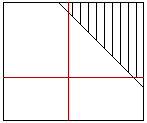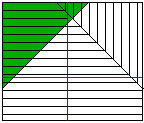Robert
5/17/96

How do you graph the inequalities

5+g<h
6-h>g
g+h>6

on one coordinate plane

Hi Robert
 To graph the inequality 5+ggGraph of the inequality g+h>6. Here are all three graphs on one coordinate plane. Notice that there are no points that satisfy all there inequalities.Graph of all three inequalities.
Cheers,
Harley and Maxine

Go to Math Central